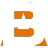# Blend or layer images

## Compositing methodsOriginal image AOriginal image BA over BA under BA atop BA inside BA outside BA screen-ed over B. “Saturating add”. Screening brightens the second image based on the luminosity of the first image. Where the first image is black, the second image will not be brightened at all. Where the first image is white, the second image will be white. Screen does not work well with HDR images.A add-ed to B. This simply adds the color values of the two images together.A subtract-ed from B. This simply subtracts the color values of A from B.A diff B This takes the absolute value of subtracting A from B.A multiply by B. This simply multiplies the color values of A and B. Multiplying with a grayscale matte is the same as masking .A min B Takes the minimum of A and B.A max B Takes the maximum of A and B.A average B Takes the average of A and B.A Xor B The union of A and B, except where A and B overlap.